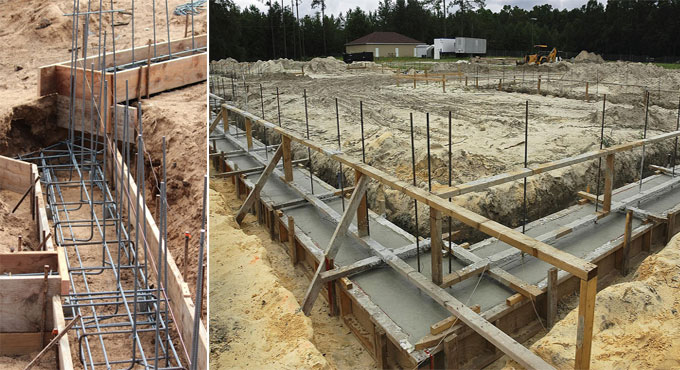# Estimation and calculation of concrete volume for Building footingsTo analyse the footings of a building one need to calculate the concrete volume first. Whether you are charting to cast the footings manually or you are using a ready mix concrete, the first and the foremost task one needs to know the physical volume of the apartment or building. Once the project plan is strategized and the concrete volume is detected, the extra materials for footing casting can be cut out.

Once you initiate the construction project, try to use the concrete made on the site using concrete mixers as it improves ductility of to the building. The extra ingredients can be used for some other amendable activities.

Once the construction plan is completed and the volume is detected, the next important thing to do is to calculate the exact amount of ready mix concrete required. Once the exact requirement of concrete mix is determined the construction project may be ready for operation.

## Steps To Calculate The Concrete Volume Of Different Footings

Once the project is planned measuring the concrete footings is easy. The steps described below can show to how to calculate the concrete volume of different footings.

One just needs to look into the drawings first as specified by the client. The designing team as well as the engineering team needs to inspect the specifications and work together.

The engineering team needs to get the volume of different sizes of footings. Then once they determine it ,one needs to summarise these footings like FI, F2, F3, F4.

For Example
F1 -5 Nos
F2 - 6 Nos
F3 - 4 Nos

### Creating Checklist And Marking The Footing After Counting

Now create a checklist by marking the footing after counting. It makes the calculation smoother.Round off one after one when it?s counted.

Check the footing lay out again and again and see anything that has been skipped.

If any footing is found unmarked or skipped, mark that too and then sum up different types of footings , and once you compiled it together calculate the volume of each specific footing by applying the geometric formula.

### Calculation Of 3D Shapes

If a 3D shape has been implemented on the construction project, the designing team has to recreate the 3D Shape which can be rectangular, square or trapezoidal and deliver it to the engineering team as per the client specifications, then the Engineering team needs to specify the volume of the 3D shapes.

For example F1 is a square size footing and the dimension will be 4x4x1 =16, So the concrete volume is 16 cubic ft, and as we know.

F1 - 6 Nos of footings,
So the total concrete volume for F1 type is.
= 5 x 16
= 80 cubic feet.

For example if it is a rectangle find out the surface area of the slab and then multiply with the depth and thickness of the slab.

Or we can calculate it in this way for example the F2 footing is a rectangular shape and the dimension is 4? x 6? x1?.

So the concrete volume for one footing is 24 cubic feet.

As we have 6 Nos of F2 footing, so the total volume for this type of footing is
= 6 x 24
= 144 cubic feet.

### Calculation Of Trapezoidal

F3 has a footing type which is Trapezoidal in shape and the calculated volume for one footing is 30 cubic ft.

And as we know F3 has 4 nos
So the total concrete volume for F3 Type is
= 4 x 30
= 120 cubic feet.

Now if we calculate the total concrete footings for our building project is
F1 +F2+ F3
= 80+144+120
= 344 cubic feet.

Thus we conclude that this is the exact estimation of the footing calculated for our building project which we have taken as an example, however the calculations may vary individually but this is the most uncomplicated and manageable calculations one can ever achieve while measuring the footing.